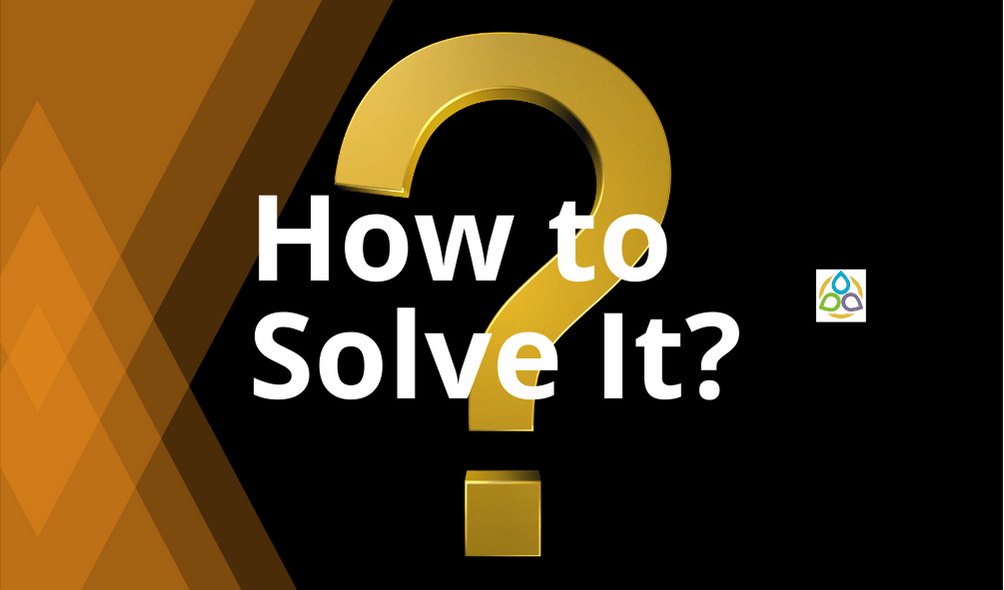# How to Solve It?

Solve. Nine square tiles are laid out on a table so that they make a solid pattern. Each tile must touch at least one other tile along an entire edge (see the following figure).

(a) What are the possible perimeters of the figures that can be formed?

(b) Which figure has the least perimeter?

Describe the strategy you used to find your solution(s).

See here.

(a) Perimeters range from 12 units to 20 units. Note that, the perimeters must be even.

(b) The figure with the least perimeter is a 3 × 3 square.

1.Shachindra says:

1 3 5 horizontal
2 3 4 vertically. And vice versa

3 is common and sum is rest two is same both ways.

1.Math1089 says:

Thank you so much for your solution. However, a complete solution is already provided, which includes your one.

2.Roger says:

2+3+4=9. Therefore, wrong answer. The total should be 8.

2.DEB JYOTI MITRA says:

If x1,x2,x3,x4 &x5 are 5 numbers ;
If Any xi comes in center i=1,2,3,4,5;
Then sum of two is sum of remaining two excluding xi;
If xi=1 remainings are 2,3,4 &5 :- 2+1+5( let it be Horizontal)=3+1+4(let it be vertical);
3+1+4 ( let it be Horizontal)=2+1+5(let it be vertical);

If xi=2 remainings are 1,3,4 & 5:- Solution not possible;
If xi=3 remainings are 1,2,4 &5 :- 1+3+5(H)=2+3+4(V);
2+3+4(H)=1+3+5(V);
If xi=4 remainings are 1,2,3 & 5:- Solution not possible;
If xi=5 remainings are 1,2,3,4 – (1+5+4)(H)=(2+5+3)(V);
(2+5+3)(H)=(1+5+4)

3.Roger says:

There are two possibilities in adding the five numbers to equal eight: 1,2,5 and 1,3,4. The number common to both permutations is 1. Therefore, the number in middle can only be 1.

1.Math1089 says:

Thank you

4.Harish Kumar Sharma says:

How did you solve problem number 10?